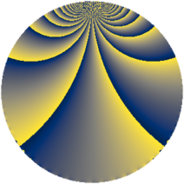# Properties

 Label 819.2.yLevel $819$ Weight $2$ Character orbit 819.y Rep. character $\chi_{819}(307,\cdot)$ Character field $\Q(\zeta_{4})$ Dimension $92$ Newform subspaces $9$ Sturm bound $224$ Trace bound $5$

# Related objects

## Defining parameters

 Level: $$N$$ $$=$$ $$819 = 3^{2} \cdot 7 \cdot 13$$ Weight: $$k$$ $$=$$ $$2$$ Character orbit: $$[\chi]$$ $$=$$ 819.y (of order $$4$$ and degree $$2$$) Character conductor: $$\operatorname{cond}(\chi)$$ $$=$$ $$91$$ Character field: $$\Q(i)$$ Newform subspaces: $$9$$ Sturm bound: $$224$$ Trace bound: $$5$$ Distinguishing $$T_p$$: $$2$$, $$5$$

## Dimensions

The following table gives the dimensions of various subspaces of $$M_{2}(819, [\chi])$$.

Total New Old
Modular forms 240 100 140
Cusp forms 208 92 116
Eisenstein series 32 8 24

## Trace form

 $$92 q + 4 q^{2} - 4 q^{8} + O(q^{10})$$ $$92 q + 4 q^{2} - 4 q^{8} - 8 q^{11} + 16 q^{14} - 112 q^{16} - 16 q^{22} + 28 q^{28} - 4 q^{29} - 24 q^{32} + 20 q^{35} - 36 q^{37} - 28 q^{44} - 40 q^{46} - 12 q^{50} + 68 q^{53} - 12 q^{58} + 24 q^{65} - 20 q^{67} - 20 q^{70} - 24 q^{71} + 80 q^{74} + 28 q^{79} - 100 q^{85} - 84 q^{86} - 16 q^{91} - 200 q^{92} - 92 q^{98} + O(q^{100})$$

## Decomposition of $$S_{2}^{\mathrm{new}}(819, [\chi])$$ into newform subspaces

Label Dim $A$ Field CM Traces $q$-expansion
$a_{2}$ $a_{3}$ $a_{5}$ $a_{7}$
819.2.y.a $4$ $6.540$ $$\Q(i, \sqrt{6})$$ None $$0$$ $$0$$ $$-8$$ $$0$$ $$q+\beta _{1}q^{2}+\beta _{2}q^{4}+(-2+2\beta _{2})q^{5}+\cdots$$
819.2.y.b $4$ $6.540$ $$\Q(i, \sqrt{10})$$ None $$0$$ $$0$$ $$-4$$ $$-4$$ $$q+2\beta _{2}q^{4}+(-1+\beta _{1}-\beta _{2})q^{5}+(-1+\cdots)q^{7}+\cdots$$
819.2.y.c $4$ $6.540$ $$\Q(i, \sqrt{10})$$ None $$0$$ $$0$$ $$4$$ $$-4$$ $$q+2\beta _{2}q^{4}+(1+\beta _{1}+\beta _{2})q^{5}+(-1+\cdots)q^{7}+\cdots$$
819.2.y.d $4$ $6.540$ $$\Q(i, \sqrt{6})$$ None $$0$$ $$0$$ $$8$$ $$4$$ $$q+\beta _{1}q^{2}+\beta _{2}q^{4}+(2-2\beta _{2})q^{5}+(1+\cdots)q^{7}+\cdots$$
819.2.y.e $8$ $6.540$ 8.0.$$\cdots$$.3 None $$0$$ $$0$$ $$0$$ $$4$$ $$q-\beta _{5}q^{2}+\beta _{2}q^{4}+(-\beta _{2}+\beta _{6})q^{7}+\cdots$$
819.2.y.f $12$ $6.540$ $$\mathbb{Q}[x]/(x^{12} + \cdots)$$ None $$0$$ $$0$$ $$-12$$ $$-4$$ $$q-\beta _{8}q^{2}+(3\beta _{4}-\beta _{6}-\beta _{11})q^{4}+(-1+\cdots)q^{5}+\cdots$$
819.2.y.g $12$ $6.540$ $$\mathbb{Q}[x]/(x^{12} + \cdots)$$ None $$0$$ $$0$$ $$12$$ $$12$$ $$q-\beta _{8}q^{2}+(3\beta _{4}-\beta _{6}-\beta _{11})q^{4}+(1+\cdots)q^{5}+\cdots$$
819.2.y.h $12$ $6.540$ $$\mathbb{Q}[x]/(x^{12} + \cdots)$$ None $$4$$ $$0$$ $$0$$ $$-8$$ $$q-\beta _{7}q^{2}+(\beta _{4}-\beta _{6}-\beta _{7}-\beta _{8})q^{4}+\cdots$$
819.2.y.i $32$ $6.540$ None $$0$$ $$0$$ $$0$$ $$0$$

## Decomposition of $$S_{2}^{\mathrm{old}}(819, [\chi])$$ into lower level spaces

$$S_{2}^{\mathrm{old}}(819, [\chi]) \cong$$ $$S_{2}^{\mathrm{new}}(91, [\chi])$$$$^{\oplus 3}$$$$\oplus$$$$S_{2}^{\mathrm{new}}(273, [\chi])$$$$^{\oplus 2}$$